Home

# ON A LATTICE CHARACTERISATION OF FINITE SOLUBLE PST-GROUPS

## Abstract

Let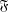$\mathfrak{F}$ be a class of finite groups and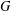$G$ a finite group. Let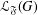${\mathcal{L}}_{\mathfrak{F}}(G)$ be the set of all subgroups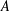$A$ of$G$ with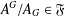$A^{G}/A_{G}\in \mathfrak{F}$ . A chief factor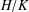$H/K$ of$G$ is$\mathfrak{F}$ -central in$G$ if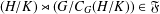$(H/K)\rtimes (G/C_{G}(H/K))\in \mathfrak{F}$ . We study the structure of$G$ under the hypothesis that every chief factor of$G$ between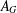$A_{G}$ and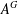$A^{G}$ is$\mathfrak{F}$ -central in$G$ for every subgroup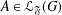$A\in {\mathcal{L}}_{\mathfrak{F}}(G)$ . As an application, we prove that a finite soluble group$G$ is a PST-group if and only if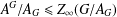$A^{G}/A_{G}\leq Z_{\infty }(G/A_{G})$ for every subgroup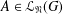$A\in {\mathcal{L}}_{\mathfrak{N}}(G)$ , where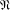$\mathfrak{N}$ is the class of all nilpotent groups.

## Footnotes

Hide All

Research of the first author is supported by the China Scholarship Council and NNSF of China (11771409).

## References

Hide All
MathJax
MathJax is a JavaScript display engine for mathematics. For more information see http://www.mathjax.org.

# ON A LATTICE CHARACTERISATION OF FINITE SOLUBLE PST-GROUPS

## Metrics

### Full text viewsFull text views reflects the number of PDF downloads, PDFs sent to Google Drive, Dropbox and Kindle and HTML full text views.

Total number of HTML views: 0
Total number of PDF views: 0 *Loading metrics...

### Abstract viewsAbstract views reflect the number of visits to the article landing page.

Total abstract views: 0 *Loading metrics...

* Views captured on Cambridge Core between <date>. This data will be updated every 24 hours.

Usage data cannot currently be displayed.